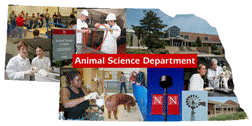## Animal Science Department## Faculty Papers and Publications in Animal Science

#### Date of this Version

January 1985

Published in J Dairy Sci1985, 68:1006-1014. Copyright © 1985 The American Dairy Science Association. Used by permission.

#### Abstract

Gauss-Seidel, successive over-relaxation, end-of-round relaxation, and block iteration methods of obtaining solutions for sire effects from equations rising from progeny with records in mixed model procedures were compared. Equations transformed to provide direct solutions for genetic group plus sire effects as well as constrained and unconstrained equations were compared also. Equations for milk yield for the Northeast Artificial Insemination Sire Comparison numbered 301 for Ayrshires, 325 for Brown Swiss, 6,010 for Holsteins, and 926 for Jerseys after absorption of herd-year-season effects. Numbers of coefficients were 15 to 20% less for transformed equations, which decreased computing time per round of interation about 15%. Solutions for transformed equations converged more rapidly than solutions for untransformed equations with convergence criterion the ratio of the residual norm to the norm of the right-hand sides. Successive over-relaxation generally was more efficient than Gauss-Seidel iteraton. Solutions for equations constrained to full rank converged more slowly than unconstrained equations. Block iteration was more efficient than single equation iteration.

COinS